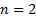/

### Probability distributions

The Nematrian website contains information and analytics on a wide range of probability distributions, including:

Discrete (univariate) distributions

Continuous (univariate) distributions

-          Beta

-          Burr

-          F

-          Fatigue, also known as the Birnbaum-Saunders distribution

-          Generalised Pareto (GDP)

-          Lévy

-          Rice

Continuous multivariate distributions

Copulas (a copula is a special type of continuous multivariate distribution)

-       Countermonotonicity (only valid for, whereis the dimension of the input)

-          t

The Nematrian website functions for fitting univariate distributions, creating random variates and calculating moments etc. now cover most of the above probability distributions, see ProbabilityDistributionsFunctions. In many cases these functions can cater for the traditional (textbook) forms of these distributions and variants that include additional shift and scale parameters.